Next: The incompleteness theorems Up: Logical paradoxesGödel's theorems, Previous: Logical paradoxesGödel's theorems,

It has precursors, but Russell's paradox of 1901 shows that the obvious set theory, as proposed by Frege has to be modified in unpleasant ways. Frege's basic idea is to let us define the set of all objects having a given property, in more modern notation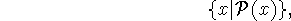giving the set of all x with the property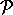. Thus the set of all red dogs is denoted by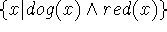, or if the set of dogs is denoted dogs and the set of red objects as reds, we can also write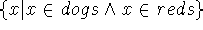. This notation for forming sets is very convenient and is much used in mathematics. The principle is called comprehension.

Bertrand Russell in his 1901 letter to Gottlob Frege pointed out that forming the set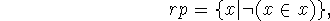i.e. the set of all sets that are not members of themselves, leads promptly to a contradiction. We get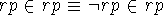.

There are many ways of restricting set theory to avoid the contradiction. The most commonly chosen is that of Zermelo, whose set theory Z allowed only writing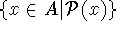, where A is a previously defined set. This turned out to be not quite enough to represent mathematics and Fraenkel introduce a further axiom schema of replacement giving a system now called ZF.

ZF is less convenient than Frege's inconsistent system because of the need to find the set A, and the unrestricted comprehension schema is often used when it is clear that the needed A could be found.A more direct inconvenience for giving robots consciousness is the paradox discovered by Richard Montague [Montague, 1963] concerning a set of desirable axioms for knowledge of sentences.

We might denote by knows(person,sentence) the assertion that person knows sentence and consider this as holding at some time t in in some situation s. However, Montague's paradox arises even when there is only one knower, and we write Kp for the knower knowing the sentence p. Montague's paradoxes arise under the assumption that the language of the sentences p is rich enough for ``elementary syntax'', i.e. allows quantifiers and operations on sentences or on Gödel numbers standing for sentences.

The axioms are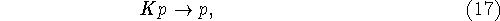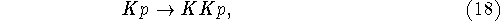and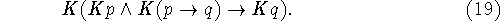Intuitively these axioms state that if you know something, it's true, if you know something, you know you know it, and you can do modus ponens. Added to this are schemas saying that you know some sentences of elementary logic.

From these, Montague constructed a version of the paradox of the liar. Hence they must be weakened, and there are many weakenings that restore consistency. Montague preferred to leave out elementary syntax, thus getting a form of modal logic.

I think it might be better to weaken (18) by introducing a hierarchy of introspective knowledge operators on the idea that knowing that you know something is knowledge at an introspective level.

Suppose that we regard knowledge as a function of time or of the situation. We can slither out of Montague's paradox by changing the axiom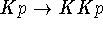to say that if you knew something in the past, you now know that you knew it. This spoils Montague's recursive construction of the paradox.

None of this has yet been worked out for an AI system.Next: The incompleteness theorems Up: Logical paradoxesGödel's theorems, Previous: Logical paradoxesGödel's theorems,

John McCarthy
Mon Jul 15 13:06:22 PDT 2002